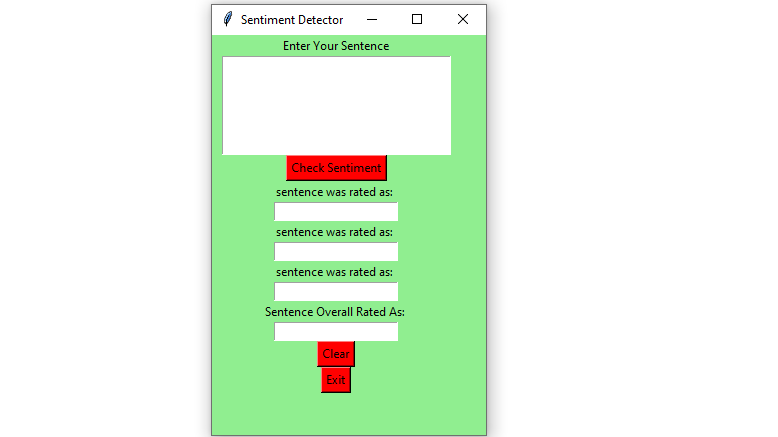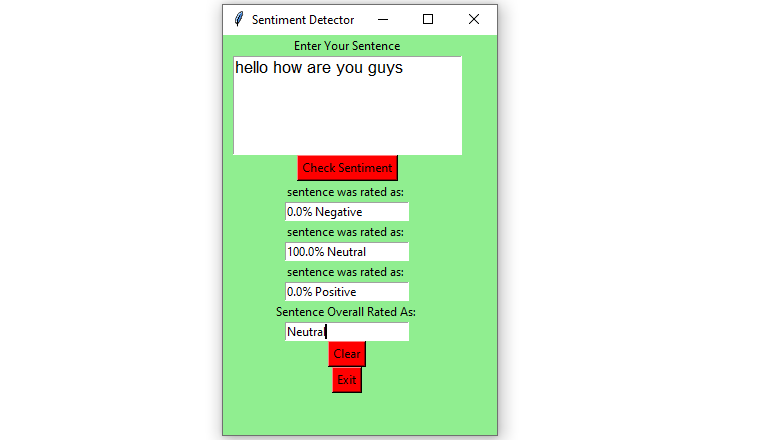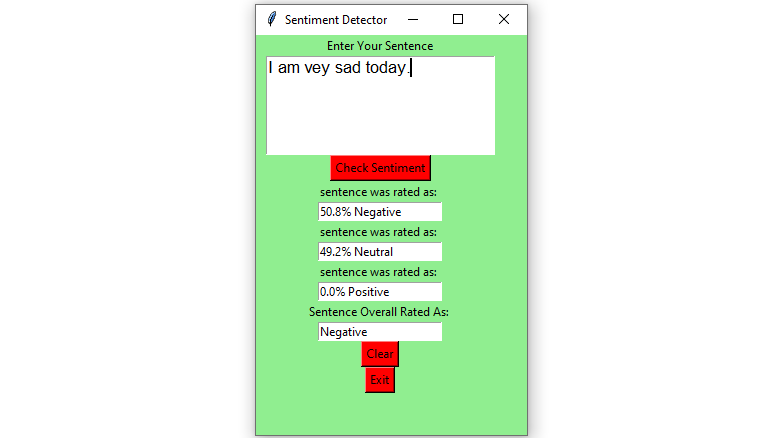# Sentiment Detector GUI using Tkinter – Python

Prerequisites : Introduction to tkinter | Sentiment Analysus using Vader

Python offers multiple options for developing a GUI (Graphical User Interface). Out of all the GUI methods, tkinter is the most commonly used method. Python with tkinter outputs the fastest and easiest way to create GUI applications. In this article, we will learn how to create a Sentiment Detector GUI application using Tkinter, with a step-by-step guide.

To create a tkinter :

• Importing the module – tkinter
• Create the main window (container)
• Add any number of widgets to the main window.
• Apply the event Trigger on the widgets.

Let’s create a GUI based Sentiment Detector application that can show the sentiments with respect to the given sentences, given by the user.

Below is the implementation :

 `# import SentimentIntensityAnalyzer class  ` `# from vaderSentiment.vaderSentiment module.  ` `from` `vaderSentiment.vaderSentiment ``import` `SentimentIntensityAnalyzer  ` ` `  `# import all methods and classes from the tkinter  ` `from` `tkinter ``import` `*` ` `  `# Function for clearing the    ` `# contents of all entry boxes ` `# And text area. ` `def` `clearAll() :  ` `   `  `    ``# deleting the content from the entry box  ` `    ``negativeField.delete(``0``, END)  ` `    ``neutralField.delete(``0``, END)  ` `    ``positiveField.delete(``0``, END)  ` `    ``overallField.delete(``0``, END)  ` ` `  `    ``# whole content of text area  is deleted  ` `    ``textArea.delete(``1.0``, END) ` `     `  `# function to print sentiments  ` `# of the sentence.  ` `def` `detect_sentiment(): ` ` `  `    ``# get a whole input content from text box ` `    ``sentence ``=` `textArea.get(``"1.0"``, ``"end"``) ` ` `  `    ``# Create a SentimentIntensityAnalyzer object.  ` `    ``sid_obj ``=` `SentimentIntensityAnalyzer()  ` ` `  `    ``# polarity_scores method of SentimentIntensityAnalyzer  ` `    ``# oject gives a sentiment dictionary.  ` `    ``# which contains pos, neg, neu, and compound scores.  ` `    ``sentiment_dict ``=` `sid_obj.polarity_scores(sentence)  ` ` `  `    ``string ``=` `str``(sentiment_dict[``'neg'``]``*``100``) ``+` `"% Negative"` `    ``negativeField.insert(``10``, string) ` `     `  ` `  `    ``string ``=` `str``(sentiment_dict[``'neu'``]``*``100``) ``+` `"% Neutral"` `    ``neutralField.insert(``10``, string) ` ` `  `    ``string ``=` `str``(sentiment_dict[``'pos'``]``*``100``) ``+``"% Positive"` `    ``positiveField.insert(``10``, string) ` `     `  `    ``# decide sentiment as positive, negative and neutral  ` `    ``if` `sentiment_dict[``'compound'``] >``=` `0.05` `: ` `        ``string ``=` `"Positive"` ` `  `    ``elif` `sentiment_dict[``'compound'``] <``=` `-` `0.05` `: ` `        ``string ``=` `"Negative"` `      `  ` `  `    ``else` `: ` `        ``string ``=` `"Neutral"` ` `  `    ``overallField.insert(``10``, string) ` `         `  ` `  ` `  `# Driver Code  ` `if` `__name__ ``=``=` `"__main__"` `: ` `     `  ` `  `    ``# Create a GUI window  ` `    ``gui ``=` `Tk()  ` `     `  `    ``# Set the background colour of GUI window  ` `    ``gui.config(background ``=`  `"light green"``)  ` ` `  `    ``# set the name of tkinter GUI window  ` `    ``gui.title(``"Sentiment Detector"``)  ` ` `  `    ``# Set the configuration of GUI window  ` `    ``gui.geometry(``"250x400"``)  ` ` `  `    ``# create a label : Enter Your Task  ` `    ``enterText ``=` `Label(gui, text ``=` `"Enter Your Sentence"``, ` `                                     ``bg ``=` `"light green"``) ` ` `  `    ``# create a text area for the root  ` `    ``# with lunida 13 font  ` `    ``# text area is for writing the content  ` `    ``textArea ``=` `Text(gui, height ``=` `5``, width ``=` `25``, font ``=` `"lucida 13"``) ` ` `  `    ``# create a Submit Button and place into the root window  ` `    ``# when user press the button, the command or   ` `    ``# function affiliated to that button is executed   ` `    ``check ``=` `Button(gui, text ``=` `"Check Sentiment"``, fg ``=` `"Black"``,  ` `                         ``bg ``=` `"Red"``, command ``=` `detect_sentiment) ` ` `  `    ``# Create a negative : label  ` `    ``negative ``=` `Label(gui, text ``=` `"sentence was rated as: "``, ` `                                        ``bg ``=` `"light green"``)  ` `   `  `    ``# Create a neutral : label  ` `    ``neutral ``=` `Label(gui, text ``=` `"sentence was rated as: "``,  ` `                                       ``bg ``=` `"light green"``)  ` `   `  `    ``# Create a positive : label  ` `    ``positive ``=` `Label(gui, text ``=` `"sentence was rated as: "``, ` `                                        ``bg ``=` `"light green"``) ` ` `  `    ``# Create a overall : label  ` `    ``overall ``=` `Label(gui, text ``=` `"Sentence Overall Rated As: "``, ` `                                           ``bg ``=` `"light green"``) ` ` `  `    ``# create a text entry box   ` `    ``negativeField ``=` `Entry(gui) ` ` `  `    ``# create a text entry box   ` `    ``neutralField ``=` `Entry(gui) ` ` `  `    ``# create a text entry box   ` `    ``positiveField ``=` `Entry(gui) ` ` `  `    ``# create a text entry box   ` `    ``overallField ``=` `Entry(gui)  ` ` `  `    ``# create a Clear Button and place into the root window  ` `    ``# when user press the button, the command or   ` `    ``# function affiliated to that button is executed .  ` `    ``clear ``=` `Button(gui, text ``=` `"Clear"``, fg ``=` `"Black"``,  ` `                      ``bg ``=` `"Red"``, command ``=` `clearAll) ` `     `  `    ``# create a Exit Button and place into the root window  ` `    ``# when user press the button, the command or   ` `    ``# function affiliated to that button is executed .  ` `    ``Exit ``=` `Button(gui, text ``=` `"Exit"``, fg ``=` `"Black"``,  ` `                        ``bg ``=` `"Red"``, command ``=` `exit) ` ` `  `    ``# grid method is used for placing   ` `    ``# the widgets at respective positions   ` `    ``# in table like structure.  ` `    ``enterText.grid(row ``=` `0``, column ``=` `2``) ` `     `  `    ``textArea.grid(row ``=` `1``, column ``=` `2``, padx ``=` `10``, sticky ``=` `W) ` `     `  `    ``check.grid(row ``=` `2``, column ``=` `2``) ` `     `  `    ``negative.grid(row ``=` `3``, column ``=` `2``) ` `     `  `    ``neutral.grid(row ``=` `5``, column ``=` `2``) ` `     `  `    ``positive.grid(row ``=` `7``, column ``=` `2``) ` `     `  `    ``overall.grid(row ``=` `9``, column ``=` `2``) ` `     `  `    ``negativeField.grid(row ``=` `4``, column ``=` `2``) ` ` `  `    ``neutralField.grid(row ``=` `6``, column ``=` `2``) ` `                       `  `    ``positiveField.grid(row ``=` `8``, column ``=` `2``) ` `     `  `    ``overallField.grid(row ``=` `10``, column ``=` `2``) ` `     `  `    ``clear.grid(row ``=` `11``, column ``=` `2``) ` `     `  `    ``Exit.grid(row ``=` `12``, column ``=` `2``) ` ` `  `    ``# start the GUI  ` `    ``gui.mainloop()  ` `    `

Output :My Personal Notes arrow_drop_upCheck out this Author's contributed articles.

If you like GeeksforGeeks and would like to contribute, you can also write an article using contribute.geeksforgeeks.org or mail your article to contribute@geeksforgeeks.org. See your article appearing on the GeeksforGeeks main page and help other Geeks.

Please Improve this article if you find anything incorrect by clicking on the "Improve Article" button below.

Article Tags :

Be the First to upvote.

Please write to us at contribute@geeksforgeeks.org to report any issue with the above content.